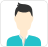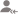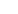true

Find the best tutors and institutes for Python Training

Find Best Python Training classes

No matching category found.

No matching Locality found.

Outside India?

Search for topicsPost a Lesson

All

All

Lessons

Discussion

Lesson Posted on 16/12/2020 IT Courses/Programming Languages/Python/Python 3.7Gaurav Kumar

In response to the role of machine learning engineer and corporate trainer , Data scientist in your esteemed...

It is used for identification purpose like- x=10; where x is variable, and it can be store int type value in the x variable. Rules of identifier:- It can be started with underscore like:- _x=10 It can be created with a variable name like- x=10, y=20, gaurav=20 python=50; but according to rule variable... read more

It is used for identification purpose like- x=10; where x is variable, and it can be store int type value in the x variable.

Rules of identifier:-

1. It can be started with underscore like:- _x=10
2. It can be created with a variable name like- x=10, y=20, gaurav=20 python=50; but according to rule variable name is not predefined in python.
3. It can not begin with a special symbol like- @x=10, @y=20
5. It can not be used to reserve words and keywords as an identifier like- True=10, False=20
Dislike Bookmark

Lesson Posted on 04/08/2020 IT Courses/Programming Languages/Python IT Courses/Programming Languages/Python/Python Classes IT Courses/Programming Languages/Python/Python Beginner +4 IT Courses/Programming Languages/Python/Python Advanced IT Courses/Programming Languages/Python/Python 2.7 IT Courses/Programming Languages/Python/Python 3.4 IT Courses/Java lessI teach Pre-primary (Nursery, KG) and Primary (I - V) students. I have Industry Experience trainer...

1. Download python from python official site search "python download" in google 2. Install in your machine 3. verify using : "python --version" command 4. Write first program using notepad create a file named test.py and write the first line "print("Hello World");" Save it. 5. Run : python test.py Code... read more

3. verify using : "python --version" command

4. Write first program using notepad

create a file named test.py and write the first line "print("Hello World");"

Save it.

5. Run : python test.py

Code Examples:

# Example
print('Hello');

# How to take user input in python
name = input('whats your name ? ')
print('hi '+ name)

Dislike BookmarkFull stack developer and Software Trainer with around 10+ years

Indention error / type error
Dislike Bookmark

Looking for Python Training classes

Find best Python Training classes in your locality on UrbanPro.

FIND NOW

Answered on 20/07/2020 IT Courses/Programming Languages/Python/Python 3.8 IT CoursesPravat Kumar Mohapatra

Trainer_QA coursesManual_Automation testing (selenium java),UFT,ISTQB certification,DB Testing,Excel

Yes , you can join to IT industry. Better you can join in software Testing field.
Dislike Bookmark

Answered on 14/06/2020 IT Courses/Programming Languages/Python/Python for Machine LearningManoj T

Machine Learning enthusiast and computer programmer.

There are three main techniques: 1) Supervised learning 2) Unsupervised learning 3) Reinforcement learning
Dislike Bookmark

Lesson Posted on 08/04/2020 IT Courses/Programming Languages/Python IT Courses/Programming Languages/Python/Python Advanced IT Courses/Data ScienceRishi

import pandas as pd from datetime import datetimeimport matplotlib.dates as datesimport matplotlib.pyplot as plt def gantt_chart(df_phase): # Now convert them to matplotlib's internal format... df_phase = pd.to_datetime(df_phase, format='%Y-%m-%d %H:%M:%S.%f') df_phase = pd.to_datetime(df_phase,... read more

import pandas as pd
from datetime import datetime
import matplotlib.dates as dates
import matplotlib.pyplot as plt

def gantt_chart(df_phase):

# Now convert them to matplotlib's internal format...

df_phase['Start Time'] = pd.to_datetime(df_phase['Start Time'], format='%Y-%m-%d %H:%M:%S.%f')

df_phase['End Time'] = pd.to_datetime(df_phase['End Time'], format='%Y-%m-%d %H:%M:%S.%f')

#Convert DF columns into lists, plt will take all values in scalar or list

sdate = df_phase['Start Time'].tolist()

edate = df_phase['End Time'].tolist()

plugin = df_phase['Plugin'].tolist()

status = df_phase['Status'].tolist()

color = []

#Store colors for success and failure to differentiate

for i in range(0, len(status)):

if status[i] == 'Success':

color.append('green')

else:

color.append('red')

#Convert time to Matplotlib number format

edate, sdate = [dates.date2num(item) for item in (edate, sdate)]

ypos = range(len(plugin))

fig, ax = plt.subplots()

time_diff = edate - sdate

# Plot the data, color is scalar or a list

# All are in form of list

ax.barh(ypos, time_diff, left=sdate, linewidth = 0.5, height=0.8, align='center', color=color)

plt.yticks(ypos, plugin)

plt.xticks(rotation=-20)

ax.axis('tight')

# We need to tell matplotlib that these are dates...

ax.xaxis_date()

plt.ylabel('Plugins', fontsize='medium', stretch = 'normal')

# bbox_inches='tight' will prevent cutting of y-label

fig.savefig(image_file, bbox_inches='tight')

plt.show()

data = [['A', '2019-06-27 18:33:58.033', '2019-06-27 19:54:04.658', 'Success'], ['B', '2019-06-27 19:54:04.957', '2019-06-27 19:58:14.570', 'Success'], ['C', '2019-06-27 19:54:04.963', '2019-06-27 19:54:19.928', 'Failed']]

df_phase = pd.DataFrame(data, columns = [Plugin, 'Start Time', 'End Time', 'Status'])

#Calling the function

gantt_chart(df_phase)

Note: This is authored my me only, you can find it on my blog.

Dislike Bookmark

Answered on 04/06/2020 Tuition/BTech Tuition IT Courses/Programming Languages/Python IT Courses/Programming Languages/Python/Python Anaconda +1 IT Courses/Programming Languages/Python/Python Advanced lessPiyush Gupta

Build Yourself For The Future

Can't solve because product price is a part of the tuple. Since LIST IS MUTABLE so we can change its elements. We can change the whole tuple because it is an element of the list. As WKT TUPLE IS IMMUTABLE so we can't change its elements.
Dislike Bookmark

Lesson Posted on 01/04/2020 IT Courses/Programming Languages/Python/Python 3.8 IT Courses/Programming Languages/Python IT Courses/Programming Languages/Python/Python Advanced +2 IT Courses/Programming Languages/Python/Python Classes IT Courses/Programming Languages/Python/Python Beginner lessNubeEra

NubeEra E-Learning Platform is Worlds s First leading Knowledge Sharing Industry which focus on ABCD-(Analytics-...

Python can be used in any futuristics technology A= Analytics Data Science Artificial Intelligence(AI) Neural Network(NN) Natural Language Processing(NLP) Computer Vision(OpenCV) In Analytics some of the use cases are Google uses in Self-driving Car and major projects Youtube uses... read more

Python can be used in any futuristics technology

• A= Analytics
• Data Science
• Artificial Intelligence(AI)
• Neural Network(NN)
• Natural Language Processing(NLP)
• Computer Vision(OpenCV)
• In Analytics some of the use cases are
1. Google uses in Self-driving Car and major projects
• B = Big Data
• Spark is the fastest Analytical Processing Engine which is 100X Faster then Hadoop
• PySpark market demands in jobs, projects and essential industrial tool.
• C = Cloud Computing
• AWS-Amazon Web Service uses Python to work with Microservices
• Microsoft Azure- uses Python in CLI
• Google Cloud Platform(GCP) uses Python
• D = Development / DevOps
• Professional moving from Java and another language to Python

Dislike Bookmark

Answered on 10/03/2020 IT Courses/Programming Languages/Python IT Courses/Java IT Courses/Programming Languages/PHPCharlie

In a scenario, if you are well versed in Python, but for getting an ideal job, you would likely to have to face several Python interview questions to prove that you know how to work with it. Some of the questions are as follows: What do you understand by "Python"? Explain memory management in Python? A=10.20,30,... read more

In a scenario, if you are well versed in Python, but for getting an ideal job, you would likely to have to face several Python interview questions to prove that you know how to work with it. Some of the questions are as follows:

What do you understand by "Python"?

Explain memory management in Python?

A=10.20,30, In this operation, what is the data type of 'A' is a tuple. When you print 'A', that Python assumes as?

How ill you get to know the version of Python you are using?

Explain the terms *args & **kwargs? And why would we use them?

These are some of the questions that might come up in your interview.

All the Best

Dislike Bookmark

Looking for Python Training classes

Find best Python Training classes in your locality on UrbanPro.

FIND NOW

Lesson Posted on 30/03/2017 IT Courses/Programming Languages/Python Tuition/BTech TuitionSri Vallabha

This is an assignment in Python, I contributed to a numerical Python MOOC from George Washington University. The link to this assignment on github is here. The Lotka–Volterra equations, also known as the predator–prey equations, are a pair of first-order, non-linear, differential equations.... read more

This is an assignment in Python, I contributed to a numerical Python MOOC from George Washington University. The link to this assignment on github is here.

The Lotka–Volterra equations, also known as the predator–prey equations, are a pair of first-order, non-linear, differential equations. They are frequently used to describe the dynamics of biological systems in which two species interact, one as a predator and the other as prey. You can read more about this from Wikipedia http://en.wikipedia.org/wiki/Lotka-Volterra_equation.

### Equations describing the system

Their populations change with time according to the following pair of equations:Where,is the number of prey (say rabbits),is the number of predators (say foxes).gives the rate of change of their respective populations over time.are the parameters describing the interaction between the two species. This models makes some assumptions about the predator and prey. You can read about them from the Wikipedia page mentioned above.

The above equations can be written in a slightly different form to interpret the physical meaning of the four parameters used.

1.Equation for preyThe prey are supposed to have unlimited supply of food andrepresents the rate of population growth of prey. Rate of decrease of population of prey is assumed to be proportional to the rate at which predator and prey meet and is given by2.Equation for predatorFor the predators,gives the rate of growth of predator population. Note that this is similar to the rate of decrease of population of prey. The second termgives the rate of population decrease for predators due to natural death or emigration.

### Numerical solution using Python

A simple python code for solving these equations is shown below.

# importrequired libraries
import numpy
import matplotlib.pyplot as plt
%matplotlib inline
# set the initial parameters
alpha = 1.
beta = 1.2
gamma = 4.
delta = 1.

#define the time stepping scheme - euler forward, as used in earlier lessons
def euler_step(u, f, dt):
"""Returns the solution at the next time-step using Euler's method.

Parameters
----------
u : array of float
solution at the previous time-step.
f : function
function to compute the right hand-side of the system of equation.
dt : float
time-increment.

Returns
-------
u_n_plus_1 : array of float
approximate solution at the next time step.
"""

return u + dt * f(u)

# define the function that represents the Lotka-Volterra equations
def f(u):
"""Returns the rate of change of species numbers.

Parameters
----------
u : array of float
array containing the solution at time n.

Returns
-------
dudt : array of float
array containing the RHS given u.
"""
x = u
y = u
return numpy.array([x*(alpha - beta*y), -y*(gamma - delta*x)])
# set time-increment and discretize the time
T  = 15.0                           # final time
dt = 0.01                           # set time-increment
N  = int(T/dt) + 1                  # number of time-steps
x0 = 10.
y0 = 2.
t0 = 0.

# set initial conditions
u_euler = numpy.empty((N, 2))

# initialize the array containing the solution for each time-step
u_euler = numpy.array([x0, y0])

# use a for loop to call the function rk2_step()
for n in range(N-1):

u_euler[n+1] = euler_step(u_euler[n], f, dt)
time = numpy.linspace(0.0, T,N)
x_euler = u_euler[:,0]
y_euler = u_euler[:,1]
plt.plot(time, x_euler, label = 'prey ')
plt.plot(time, y_euler, label = 'predator')
plt.legend(loc='upper right')
#labels
plt.xlabel("time")
plt.ylabel("number of each species")
#title
plt.title("predator prey model")
Dislike Bookmark

UrbanPro.com helps you to connect with the best Python Training classes in India. Post Your Requirement today and get connected.

Overview

Questions 1.2 k

Lessons 83

Total Shares91,991 Followers

## Python Training in:

x

X

### Looking for Python Training Classes?

Find best tutors for Python Training Classes by posting a requirement.

• Post a learning requirement
• Get customized responses
• Compare and select the best### Looking for Python Training Classes?

Find best Python Training Classes in your locality on UrbanProUrbanPro.com is India's largest network of most trusted tutors and institutes. Over 55 lakh students rely on UrbanPro.com, to fulfill their learning requirements across 1,000+ categories. Using UrbanPro.com, parents, and students can compare multiple Tutors and Institutes and choose the one that best suits their requirements. More than 7.5 lakh verified Tutors and Institutes are helping millions of students every day and growing their tutoring business on UrbanPro.com. Whether you are looking for a tutor to learn mathematics, a German language trainer to brush up your German language skills or an institute to upgrade your IT skills, we have got the best selection of Tutors and Training Institutes for you. Read more